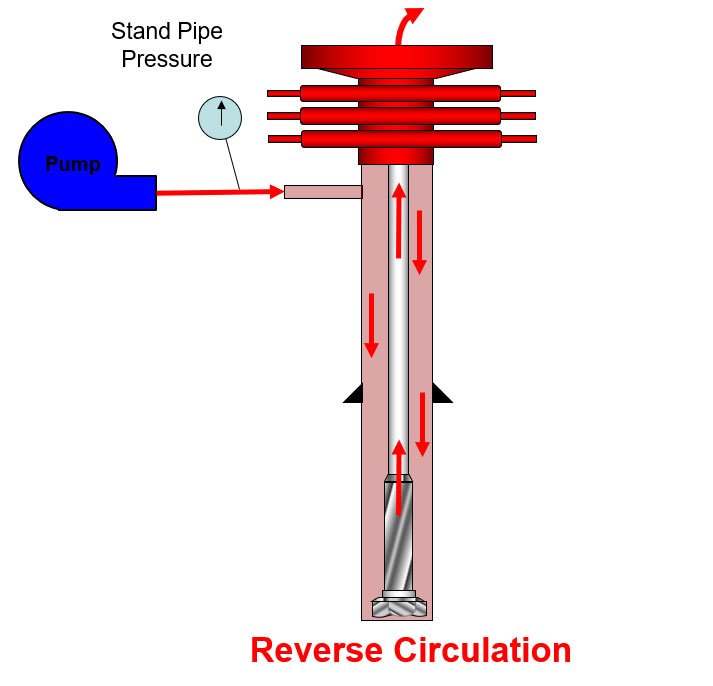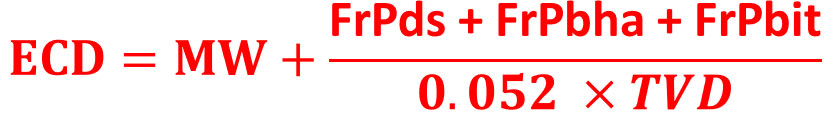# Effect of Frictional Pressure on ECD while Reverse circulation

A reverse circulation is another way to circulate by circulating into annulus up to a bit and drill string. The fluid outlet is on surface. For drilling operation, we most of the time use forward circulation; however, in completion operation, the reverse circulation is utilized more often. For more understanding, we would like to show an image below (Figure 1) which demonstrates a flow path of the reverse circulation.Figure 1 – Reverse Circulation

In this article, it will demonstrate the effect of frictional pressure on the bottom hole pressure and equivalent circulating density while the reverse circulation is performed.

## Under a static condition:

The bottom hole pressure is equal to hydrostatic pressure from the drilling fluid.

Bottom Hole Pressure (BHP)  = Hydrostatic Pressure (HP)

## Under a dynamic condition:

Stand pipe pressure equates to summation of pressure loss of whole system.Figure 2 – Pressure loss component during reverse circulation

SPP = FrPds + FrPbha + FrPbit + FrPann

Where;

SPP = Stand Pipe Pressure

FrPds = Pressure loss in drill string

FrPbha = Pressure loss in BHA

FrPbit= Pressure loss across the bit

FrPann = Pressure loss in Annulus

Please remember that the pressure loss acts opposite way while fluid is being moved.

For this case we can write the relationship of bottom hole pressure as shown in the following equation.

Bottom Hole Pressure = Hydrostatic Pressure + FrPds + FrPbha + FrPbit

Where;

FrPds = Pressure loss in drill string

FrPbha = Pressure loss in BHA

FrPbit= Pressure loss across the bit

The bottom hole pressure can be expressed in terms of the equivalent circulating density:Where;

ECD = Equivalent Circulating Density, ppg

MW = Mud Weight in the well, ppg

FrPds = Pressure loss in drill string

FrPbha = Pressure loss in BHA

FrPbit= Pressure loss across the bit

TVD = True Vertical Depth, ft

References

Cormack, D. (2007). An introduction to well control calculations for drilling operations. 1st ed. Texas: Springer.

Crumpton, H. (2010). Well Control for Completions and Interventions. 1st ed. Texas: Gulf Publishing.

Grace, R. (2003). Blowout and well control handbook [recurso electrónico]. 1st ed. Paises Bajos: Gulf Professional Pub.

Grace, R. and Cudd, B. (1994). Advanced blowout & well control. 1st ed. Houston: Gulf Publishing Company.

Watson, D., Brittenham, T. and Moore, P. (2003). Advanced well control. 1st ed. Richardson, Tex.: Society of Petroleum Engineers.

Share the joy